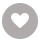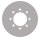# Learning pandas - Second Edition电子书

67人正在读 | 0人评论9.8

• 读书简介
• 目录
• 累计评论(0条)
• 读书简介
• 目录
• 累计评论(0条)
Get to grips with pandas—a versatile and high-performance Python library for data manipulation, analysis, and discovery About This Book ? Get comfortable using pandas and Python as an effective data exploration and analysis tool ? Explore pandas through a framework of data analysis, with an explanation of how pandas is well suited for the various stages in a data analysis process ? A comprehensive guide to pandas with many of clear and practical examples to help you get up and using pandas Who This Book Is For This book is ideal for data scientists, data analysts, Python programmers who want to plunge into data analysis using pandas, and anyone with a curiosity about analyzing data. Some knowledge of statistics and programming will be helpful to get the most out of this book but not strictly required. Prior exposure to pandas is also not required. What You Will Learn ? Understand how data analysts and scientists think about of the processes of gathering and understanding data ? Learn how pandas can be used to support the end-to-end process of data analysis ? Use pandas Series and DataFrame objects to represent single and multivariate data ? Slicing and dicing data with pandas, as well as combining, grouping, and aggregating data from multiple sources ? How to access data from external sources such as files, databases, and web services ? Represent and manipulate time-series data and the many of the intricacies involved with this type of data ? How to visualize statistical information ? How to use pandas to solve several common data representation and analysis problems within finance In Detail You will learn how to use pandas to perform data analysis in Python. You will start with an overview of data analysis and iteratively progress from modeling data, to accessing data from remote sources, performing numeric and statistical analysis, through indexing and performing aggregate analysis, and finally to visualizing statistical data and applying pandas to finance. With the knowledge you gain from this book, you will quickly learn pandas and how it can empower you in the exciting world of data manipulation, analysis and science. Style and approach ? Step-by-step instruction on using pandas within an end-to-end framework of performing data analysis ? Practical demonstration of using Python and pandas using interactive and incremental examples

Title Page

Learning pandas

Second Edition

Learning pandas

Second Edition

Credits

www.PacktPub.com

Why subscribe?

Customer Feedback

Preface

What this book covers

What you need for this book

Who this book is for

Conventions

Customer support

Errata

Piracy

Questions

pandas and Data Analysis

Introducing pandas

Data manipulation, analysis, science, and pandas

Data manipulation

Data analysis

Data science

Where does pandas fit?

The process of data analysis

The process

Ideation

Retrieval

Preparation

Exploration

Modeling

Presentation

Reproduction

A note on being iterative and agile

Relating the book to the process

Concepts of data and analysis in our tour of pandas

Types of data

Structured

Unstructured

Semi-structured

Variables

Categorical

Continuous

Discrete

Time series data

General concepts of analysis and statistics

Quantitative versus qualitative data/analysis

Single and multivariate analysis

Descriptive statistics

Inferential statistics

Stochastic models

Probability and Bayesian statistics

Correlation

Regression

Other Python libraries of value with pandas

Numeric and scientific computing - NumPy and SciPy

Statistical analysis – StatsModels

Machine learning – scikit-learn

PyMC - stochastic Bayesian modeling

Data visualization - matplotlib and seaborn

Matplotlib

Seaborn

Summary

Up and Running with pandas

Installation of Anaconda

IPython and Jupyter Notebook

IPython

Jupyter Notebook

Introducing the pandas Series and DataFrame

Importing pandas

The pandas Series

The pandas DataFrame

Visualization

Summary

Representing Univariate Data with the Series

Configuring pandas

Creating a Series

Creating a Series using Python lists and dictionaries

Creation using NumPy functions

Creation using a scalar value

The .index and .values properties

The size and shape of a Series

Specifying an index at creation

Retrieving values in a Series by label or position

Lookup by label using the [] operator and the .ix[] property

Explicit lookup by position with .iloc[]

Explicit lookup by labels with .loc[]

Slicing a Series into subsets

Alignment via index labels

Performing Boolean selection

Re-indexing a Series

Modifying a Series in-place

Summary

Representing Tabular and Multivariate Data with the DataFrame

Configuring pandas

Creating DataFrame objects

Creating a DataFrame using NumPy function results

Creating a DataFrame using a Python dictionary and pandas Series objects

Creating a DataFrame from a CSV file

Accessing data within a DataFrame

Selecting the columns of a DataFrame

Selecting rows of a DataFrame

Scalar lookup by label or location using .at[] and .iat[]

Slicing using the [ ] operator

Selecting rows using Boolean selection

Selecting across both rows and columns

Summary

Manipulating DataFrame Structure

Configuring pandas

Renaming columns

Adding new columns with [] and .insert()

Reordering columns

Replacing the contents of a column

Deleting columns

Appending new rows

Concatenating rows

Adding and replacing rows via enlargement

Removing rows using .drop()

Removing rows using Boolean selection

Removing rows using a slice

Summary

Indexing Data

Configuring pandas

The importance of indexes

The pandas index types

The fundamental type - Index

Integer index labels using Int64Index and RangeIndex

Floating-point labels using Float64Index

Representing discrete intervals using IntervalIndex

Categorical values as an index - CategoricalIndex

Indexing by date and time using DatetimeIndex

Indexing periods of time using PeriodIndex

Working with Indexes

Creating and using an index with a Series or DataFrame

Selecting values using an index

Moving data to and from the index

Reindexing a pandas object

Hierarchical indexing

Summary

Categorical Data

Configuring pandas

Creating Categoricals

Renaming categories

Appending new categories

Removing categories

Removing unused categories

Setting categories

Descriptive information of a Categorical

Summary

Numerical and Statistical Methods

Configuring pandas

Performing numerical methods on pandas objects

Performing arithmetic on a DataFrame or Series

Getting the counts of values

Determining unique values (and their counts)

Finding minimum and maximum values

Locating the n-smallest and n-largest values

Calculating accumulated values

Performing statistical processes on pandas objects

Retrieving summary descriptive statistics

Measuring central tendency: mean, median, and mode

Calculating the mean

Finding the median

Determining the mode

Calculating variance and standard deviation

Measuring variance

Finding the standard deviation

Determining covariance and correlation

Calculating covariance

Determining correlation

Performing discretization and quantiling of data

Calculating the rank of values

Calculating the percent change at each sample of a series

Performing moving-window operations

Executing random sampling of data

Summary

Accessing Data

Configuring pandas

Working with CSV and text/tabular format data

Examining the sample CSV data set

Reading a CSV file into a DataFrame

Specifying the index column when reading a CSV file

Data type inference and specification

Specifying column names

Saving DataFrame to a CSV file

Working with general field-delimited data

Handling variants of formats in field-delimited data

Reading and writing data in Excel format

Reading HTML data from the web

Reading and writing HDF5 format files

Accessing CSV data on the web

Reading and writing from/to SQL databases

Reading data from remote data services

Retrieving options data from Google Finance

Reading economic data from the Federal Reserve Bank of St. Louis

Accessing Kenneth French's data

Summary

Configuring pandas

How to work with missing data

Determining NaN values in pandas objects

Selecting out or dropping missing data

Handling of NaN values in mathematical operations

Filling in missing data

Forward and backward filling of missing values

Filling using index labels

Performing interpolation of missing values

Handling duplicate data

Transforming data

Mapping data into different values

Replacing values

Applying functions to transform data

Summary

Combining, Relating, and Reshaping Data

Configuring pandas

Concatenating data in multiple objects

Understanding the default semantics of concatenation

Switching axes of alignment

Specifying join type

Appending versus concatenation

Ignoring the index labels

Merging and joining data

Merging data from multiple pandas objects

Specifying the join semantics of a merge operation

Pivoting data to and from value and indexes

Stacking and unstacking

Stacking using non-hierarchical indexes

Unstacking using hierarchical indexes

Melting data to and from long and wide format

Performance benefits of stacked data

Summary

Data Aggregation

Configuring pandas

The split, apply, and combine (SAC) pattern

Data for the examples

Splitting data

Grouping by a single column's values

Accessing the results of a grouping

Grouping using multiple columns

Grouping using index levels

Applying aggregate functions, transforms, and filters

Applying aggregation functions to groups

Transforming groups of data

The general process of transformation

Filling missing values with the mean of the group

Calculating normalized z-scores with a transformation

Filtering groups from aggregation

Summary

Time-Series Modelling

Setting up the IPython notebook

Representation of dates, time, and intervals

The datetime, day, and time objects

Representing a point in time with a Timestamp

Using a Timedelta to represent a time interval

Introducing time-series data

Indexing using DatetimeIndex

Creating time-series with specific frequencies

Calculating new dates using offsets

Representing data intervals with date offsets

Anchored offsets

Representing durations of time using Period

Modelling an interval of time with a Period

Indexing using the PeriodIndex

Handling holidays using calendars

Normalizing timestamps using time zones

Manipulating time-series data

Shifting and lagging

Performing frequency conversion on a time-series

Up and down resampling of a time-series

Time-series moving-window operations

Summary

Visualization

Configuring pandas

Plotting basics with pandas

Creating time-series charts

Adding a title and changing axes labels

Specifying the legend content and position

Specifying line colors, styles, thickness, and markers

Specifying tick mark locations and tick labels

Formatting axes' tick date labels using formatters

Common plots used in statistical analyses

Showing relative differences with bar plots

Picturing distributions of data with histograms

Depicting distributions of categorical data with box and whisker charts

Demonstrating cumulative totals with area plots

Relationships between two variables with scatter plots

Estimates of distribution with the kernel density plot

Correlations between multiple variables with the scatter plot matrix

Strengths of relationships in multiple variables with heatmaps

Manually rendering multiple plots in a single chart

Summary

Historical Stock Price Analysis

Setting up the IPython notebook

Obtaining and organizing stock data from Google

Plotting time-series prices

Plotting volume-series data

Calculating the simple daily percentage change in closing price

Calculating simple daily cumulative returns of a stock

Resampling data from daily to monthly returns

Analyzing distribution of returns

Performing a moving-average calculation

Comparison of average daily returns across stocks

Correlation of stocks based on the daily percentage change of the closing price

Calculating the volatility of stocks

Determining risk relative to expected returns

Summary

01

### HoloLens Blueprints￥190.48

Abhijit Jana,Manish Sharma,Mallikarjuna Rao
￥190.48
02

### Introduction to JVM Languages￥190.48

Vincent van der Leun
￥190.48
03

### ArcPy and ArcGIS - Second Edition￥212.45

Silas Toms,Dara O'Beirne
￥212.45
04

Micheal Lanham
￥190.48
05

Micael DaGraça
￥212.45
06

### vSphere High Performance Cookbook - Second Edition￥212.45

Kevin Elder,Christopher Kusek,Prasenjit Sarkar
￥212.45
07

Ralph Winters
￥212.45
08

Daniel Arbuckle
￥168.50
09

### Kali Linux Network Scanning Cookbook - Second Edition￥212.45

Michael Hixon,Justin Hutchens
￥212.45
10

### Reactive Android Programming￥168.50

￥168.50
•关注我们

最受欢迎的阅读产品

关注我们：

•关于我们

欢迎反馈宝贵意见给我们

客服书吧：当当云阅读问答